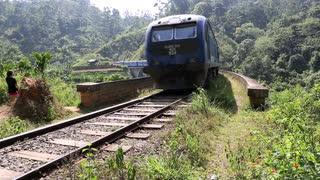# train and Bridge riddle

John walks over a railway-bridge. At the moment that he is just ten meters away from the middle of the bridge, he hears a train coming from behind. At that moment, the train, which travels at a speed of 90 km/h, is exactly as far away from the bridge as the bridge measures in length. Without hesitation, John rushes straight towards the train to get off the bridge. In this way, he misses the train by just four meters! If John had rushed exactly as fast in the other direction, the train would have hit him eight meters before the end of the bridge.

What is the length of the railway-bridge?we konw ,
speed = distance/time        & length of bridge = 2x

If john runs back , he misses the train by just 4 meters that means ,
that means (time taken by john to reach the right end of bridge) = (time taken by the train to reach 4meters before the start of bridge)
speed (train) = 90kmph = (2x – 4)/t         &          speed (john) = (x-10)/t      {refer the above diagram}
t = (2x-4)/90 = (x-10)/speed(john)   ………………………………(1)

similarly,
if john runs to the left of the bridge the train hits him 8 meters before the end so,
(time taken by the train  to reach 8 meters before left end of the bridge ) = time taken by john to reach 8 meters before the left end of the bridge)
speed(train) = 90 = (2x + 2x -8)/t*          &     speed(john) = (x -8 + 10) / t*

t* = (4x-8)/90 = (x+2)/speed(john)
so,
speed(john) = (x+2)/(4x-8)(90) ……………………………………………(2)

substitute(2) in equation (1)…..
you get the answer.
NOTE: (The units does not matter as it is going to get cancelled from both RHS and LHS)I think you did wrong

on 31st October 2018.tell me where have i gone wrong..i’ll try to rectify..

on 31st October 2018.units are not dealt with properly in calculation I think km/h and meters

on 1st November 2018.I also got the answer 44:

Bridge is B meter long
starting position John: B/2-10
starting position train: -B

situation 1: Running back
the train traveled B-4 meter
time it took: (B-4)/vt
John traveled B/2-10 meter in the same time
John’s speed vj = vt*(B/2-10)/(B-4)

situation 2: running forward
the train traveled 2B-8 (=2*(B-4)) meter
time it took: (2B-8)/vt
John traveled (B-8) – (B/2-10) = B/2+2 meter in the same time
John’s speed vj = vt*(B/2+2)/(2B-8)

vt*(B/2-10)/(B-4) = vt*(B/2+2)/(2B-8)
(B/2-10) = (B/2+2)/2
2B-40 = B+4
B = 44

vj=vt*0,3 = 27 km/h (about 17 mi/h)

all units used are meters.
The speed isn’t used at all so its units aren’t relevant

on 1st November 2018.